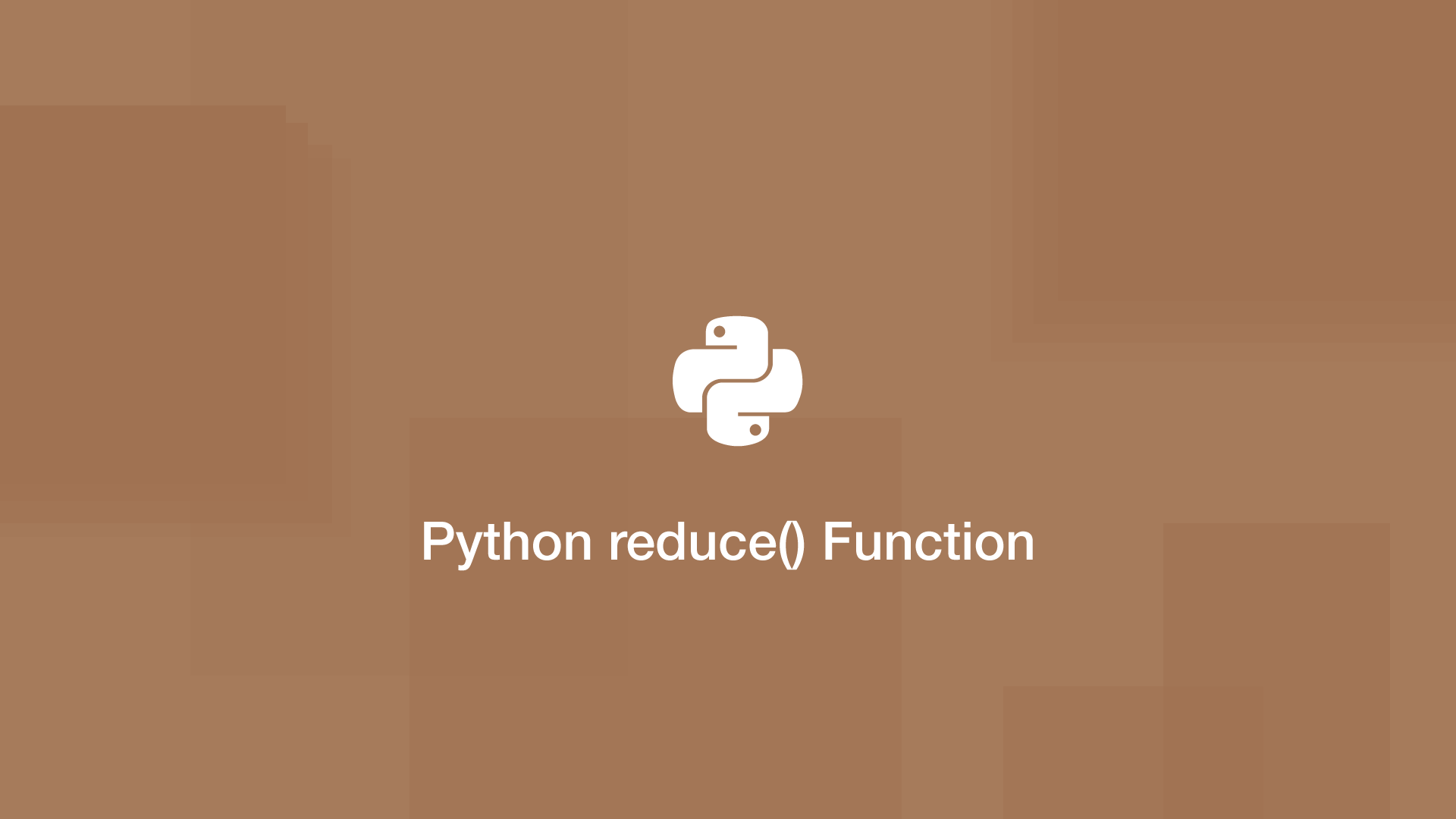# How to use the Python reduce() FunctionThe `reduce()` function in Python loops through an iterable and calls a function using each element. Each iteration takes the result from the previous function and uses it until the end of the sequence is complete.

This may sound a little confusing, but in practice is rather simple to understand how the `reduce()` function works. Let's look at the syntax of `reduce()` then at some examples.

## reduce() Syntax

The first argument of `reduce()` is the function to use, the second is the sequence. You can supply an optional start value as the third argument.

``````reduce(function, sequence[, initial])
``````

Note – If you are using Python 3, you will need to import reduce from `functools` like this:

``````from functools import reduce
``````

## Sum of All Numbers in a List

In the example below, we will get the sum of all numbers in a list using a function that accepts two arguments which will be called by `reduce()`.

``````from functools import reduce

def add_nums(a, b): return a + b

numbers = [4, 3, 7, 8, 1, 9]

print(result)
``````
``````32
``````

Here is another example demonstrating the use of `reduce()` with an initial value supplied:

``````from functools import reduce

def add_nums(a, b): return a + b

numbers = [4, 3, 7, 8, 1, 9]

print(result)
``````
``````42
``````

## Conclusion

The advantage of using the Python `reduce()` function of creating a function and performing a for loop is that it can be faster. It was moved to the `functools` module in Python 3 because it tends to only provide a clearer syntax in simple use cases.

#### Related Tutorials### How To Use Functions in Python

August 31, 2020### How to use the JavaScript reduce() Method

November 06, 2020### How to Show All Properties of an Object in Python

October 07, 2020### How to Enable Night Light Mode on Windows 10

May 16, 2020### Sum of an Array in JavaScript

September 18, 2021### How to Add Two Hex Numbers in Python

November 16, 2021